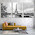Weak Acid Weak Base pH calculation solved example | Chemistry Net

# Weak Acid Weak Base pH calculation solved example

Weak Acid Weak Base pH calculation solved example

# Weak Acid Base pH calculation - Solved Example

In a previous post entitled “Weak Acids and Bases – Calculate the pH of a weak acida general equation was derived for a weak acid base pH calculation (mathematical approach). A four-step method was also proposed for weak electrolyte pH calculation (calculate the pH for a weak acid - calculate the pH for a weak base, chemical approach) (Fig. I.1).
Solved examples using the above four-step method were presented in the post “Solving Weak Acid and Weak Base pH problems
Some extra solved examples on weak acid (base) chemistry are shown below. The “chemical approach” method is used for the solution.Fig. I.1: Flowchart showing how to calculate the pH of a weak acid solution

Example I.1
Determine the pH of a 0.1 Μ weak acid HA solution (ka = 10-5). Suppose that 1 ml of this solution is added to a beaker that contains 99 ml of H2O to produce a new solution with total volume 100 ml. What is the pH value of the new solution?

 DATA [HA] = 0.1 M ka = 10-5 VH2O = 99 ml UNKNOWNS a) pH = ?  b) (pH)NEW = ;

Step 1: Write the ionization equilibrium reaction. The pH of a weak acid of known concentration has to be calculated
HA is a weak acid as the ka value shows. It dissociates partially in water according to equation (1):Step 2: Write down the equilibrium constant expression and the equilibrium constant:
ka = [Η+] . [A-] / [HA] = 10-5       (2)

Step 3:   a) Let us suppose that x M of HA dissociate. Then the equilibrium concentrations of the species involved are as follows:
 HA Η+ Α- Initial 0.1 Μ 0 Μ 0 Μ Change -x M +x M +x M Final (at equilibrium) (0.1–x) M x  M x M

b) The moles of HA nHA in the new solution can be calculated as follows:
1000 ml solution HA contains 0.1 moles «pure» ΗΑ
1  ml  solution HA contains  y = ; moles «pure» ΗΑ        y = 10-4moles «pure» ΗΑ.
The concentration of ΗΑ in the new solution can be calculated as follows:
100  ml HA contain      10-4moles «pure» ΗΑ
1000 ml  HA contain     y = ; moles «pure» ΗΑ              y = 10-3moles «pure» ΗΑ.
Therefore, the initial concentration of ΗΑ in the new solution is equal to:
[HA] = 10-3 M    (6)
From  (6) and similarly  to (α) the [Η+] concentration in the new solution can be calculated:
ka = (x). (x) /(0.001-x) = 10-5   and   x2 / 0.001 = 10-5    x = [H+] = 10-4M   (6)
Step 4:
a) From (2) and the equilibrium concentrations from the above table:
(x). (x) /(0.1-x) =  10-5    and     x2 / 0.1 = 10-5     and    x = 10-3 M   (3)
(0,1-x) ≈ x  since HA is a weak acid and dissociates slightly in water (as the ka shows).
The above assumption is valid since [HAdissociated]/ [HAinitial] * 100 = 1%  < 5%
Therefore [Η+] = [A-] = 10-3 M  (4)  and   pH = -log([H+]) = -log([10-3]) = 3
b) pH = -log([H+]) = -log([10-4]) = 4

The pH of the weak acid Ha is equal to 4 (concentration [HA] = 0.1 M)

Relevant Posts - Relevant Videos

References
1. J.N. Butler  “Ionic Equilibrium – Solubility and pH calculations”, Wiley – Interscience, 1998
2. J-L. Burgot “Ionic Equilibria in Analytical Chemistry”, Springer Science & Business Media, 2012
3. D. Harvey,  “Modern Analytical Chemistry”, McGraw-Hill Companies Inc., 2000
4. J.N. Spencer et al., “Chemistry structure and dynamics”, 5th Edition, John Wiley & Sons, Inc., 2012

Key Terms
weak acids and bases, calculate the pH of a weak acid, calculate the pH of a weak base, weak acid base chemistry

#### 1 comment:

1.In stock products can be shipped out within 3-5 business days upon receipt of customers' purchase order. SOLWAY BLUE RN